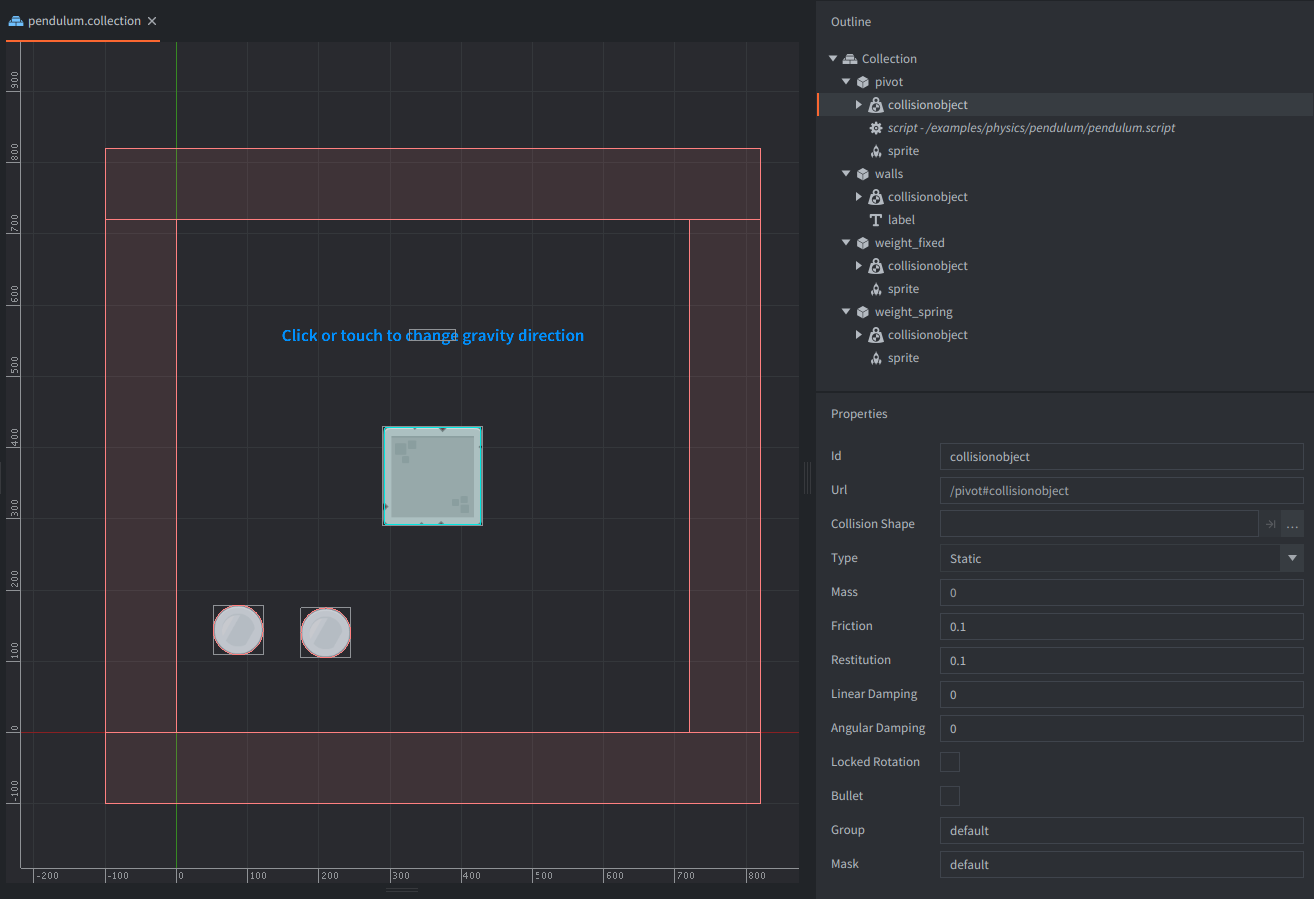#### SetupThe setup consists of four game objects. The game.project physics GravityY property is set to -500 to match the scale of the setup.

pivot
The square stone block. Contains:
• A Sprite component with the stone block image.
• A Collision object component. The Type is set to `STATIC`. A box Shape matching the sprite image is added to the components.
• A script that joines the weights game objects to to the pivot and reacts to user input by changing the direction of the physics gravity.
• A label with an instruction to the user.
weight_fixed
The cirular metal weight. Contains:
• A Sprite component with the metal circle image.
• A Collision object component. Also has Type set to `DYNAMIC`. A box Shape matching the sprite image is added to the component.
weight_spring
The same as above.
walls
The outer walls. Contains:
• A Collision object component. The Type is set to `STATIC`. 4 box Shapes are added to the component. These are placed just outside of the game view.

#### Scripts

pendulum.script

```local function draw_line(from, to)
msg.post("@render:", "draw_line", { start_point = from, end_point = to, color = vmath.vector4(1,0,0,1) }) -- <1>
end

function init(self)
msg.post(".", "acquire_input_focus") -- <2>
self.gravity = physics.get_gravity() -- <3>
self.pivot_pos = go.get_position()   -- <4>

local center_anchor = vmath.vector3(0, 0, 0) -- <5>
local pivot = "pivot#collisionobject"
local weight_fixed = "weight_fixed#collisionobject"
local weight_spring = "weight_spring#collisionobject"
physics.create_joint(physics.JOINT_TYPE_FIXED, weight_fixed, "weight_fixed_joint", center_anchor, pivot, center_anchor, {max_length = 250}) -- <6>
physics.create_joint(physics.JOINT_TYPE_SPRING, weight_spring, "weight_spring_joint", center_anchor, pivot, center_anchor, {length = 150, frequency = 1, damping = 0}) -- <7>
end

function update(self, dt)
local weight_pos = go.get_position("/weight_fixed") -- <8>
local weight1_pos = go.get_position("/weight_spring")
draw_line(self.pivot_pos, weight_pos)  -- <9>
draw_line(self.pivot_pos, weight1_pos)
end

function on_input(self, action_id, action)
if action_id == hash("touch") and action.pressed then -- <10>
if self.gravity.y ~= 0 then -- <11>
self.gravity.y = 0
self.gravity.x = 500
else
self.gravity.y = -500
self.gravity.x = 0
end
physics.set_gravity(self.gravity) -- <12>
end
end

--[[
1. Helper function to draw a line between two points.
2. Tell the engine that this object ("." is shorthand for the current game object) should listen to input. Any input will be received in the `on_input()` function.
3. Get current physics gravity vector and store it in self reference to change it later.
4. Get current position of the pivot and store it in self reference for drawing a line between the pivot and weights.
5. Store vector used for anchoring joints and collision objects ids in local variables for ease of use in below function.
6. Create a fixed joint between a first weight and the pivot
7. create a spring type joing between the second weight and the pivot.
8. Get updated positions of both weights.
9. Draw lines between the weights and the pivot.
10. If we receive input (touch or mouse click) we switch the direction of the gravity pull.
11. If the gravity is set to the bottom of the screen, set it so it pulls to the right, in other case, set it back to pull to the bottom.
12. Set the new gravity vector.
--]]
```

If you want to play with these examples, you can get the project on Github.

Do you want to see more examples? Why not write a few yourself and submit a pull request? We love contributions.